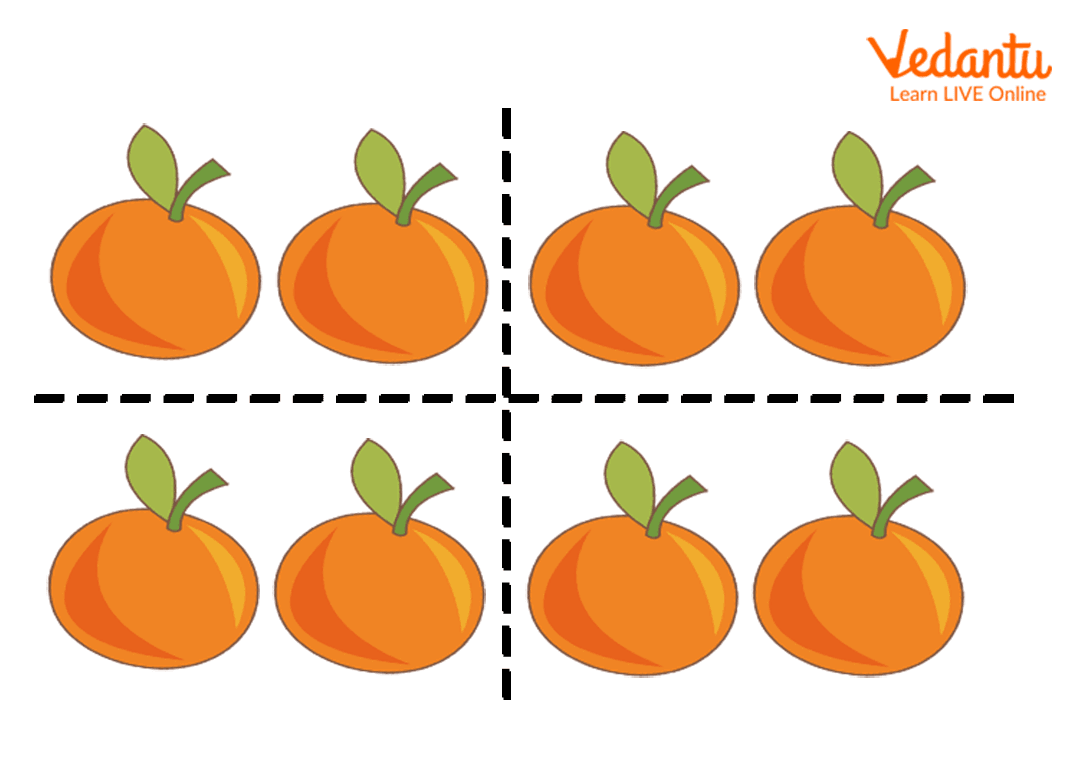Courses
Courses for Kids
Free study material
Free LIVE classes
More

# Introduction of the Quarter in Maths for KidsLIVE
Join Vedantu’s FREE Mastercalss

## Introduction of Terms Like Halves and Quarter in Maths

We generally come across the terms like half, quarter, and full as these terms are used in day-to-day life. So, it is crucial to understand the meaning of the terms. Although the terms are used commonly in daily conversation, they have a Mathematical meaning. In a more precise sense, quarters in Maths represent a quantitative value.

In this article, we aim to develop a clear understanding of the terms like half and quarter in Maths. Understanding such terms can help kids to better solve the problems in Maths. Apart from this, knowledge of such terms can also help kids to have better conversational skills.

## What are Halves and Quarters in Maths?

Halves in Maths are defined as the exact value that is obtained when the total is divided by 2. The two halves consist of exact proportions. The term quarter represents one-fourth of the total value. A quarter value can be determined by dividing the total value by four. Let us look into an example for understanding the term more precisely.

Example 1: Let us consider a girl named Rani who has 8 oranges. She was told to give a quarter of her oranges to her friend. How many oranges will Rani give to her friend?

Solution: As mentioned earlier for calculating quarters in Maths, we must divide the value by 4. Since Rani has 8 oranges, we will have to divide the oranges into 4 equal parts as shown in the image.Illustrating 8 oranges divided into 4 parts

$\frac{8}{4}$ = 2

The quarter of 8 is equal to 2; hence, Rani has to give 2 oranges to her friend. Let us look into some more examples of the calculation of quarters in Maths.

Example 2: Calculate the half and the quarter value for 20.

Solution: Half value is defined as the two equal parts, so the half of 20 can be calculated by dividing 20 by 2 whereas the quarter value of 20 can be calculated by dividing it by 4.

$\frac{20}{2}$=10, the half value of 20 is 10.

$\frac{20}{4}$, the quarter value of 20 is 5.

## Fun Fact

In an analogue watch, the term quarter is used to represent 15 minutes and seconds. For example, when someone says the time is a quarter past 6, it refers that the time is 6:15. We can tell the time, by using the terms quarter and half. Similarly, the phrase half past 6 refers to 6:30.

## Conclusion

This article includes the meaning of quarter and half in Maths. We have also learnt how to solve the questions based on the quarter and half. Understanding these is really important as it not only helps in the development of scientific thinking but also helps kids to communicate in a better way. The knowledge of these terms can help kids to adapt the society. We hope to have helped in creating a precise understanding of the term.

Last updated date: 19th Sep 2023
Total views: 131.4k
Views today: 3.31k

## FAQs on Introduction of the Quarter in Maths for Kids

1. What is the value of quarter in the phrase quarter till 6 in terms of time.

The term quarter till six indicates that there are 15 minutes left at 6 O’clock. The actual time according to the phrase is 5:45. The term quarter represents the value of 15 minutes in the case of times.

2. Give an example where the quarter is used in day-to-day conversation.

The term is used in various day-to-day conversations. It is used as a unit of measurement; for example, if a person visits a fruit market, they can calculate the price of fruit for a quarter pound of the fruit. It is also used to refer to the time, like quarter past 1 or quarter till 1.